# Voevodsky motives

• ## General Introduction

Use Friedlander's Bourbaki article.

<]]>

<]]>
• ## Online References

http://ncatlab.org/nlab/show/Voevodsky+motive

Voevodsky: Homology of schemes I. Has a really nice introduction, describing some intuition for the construction of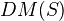, and also about the notion of universal cohomology. See section 4 for construction of DM. Possibly this is improved in later writings and/or by Déglise and Cisinski.

For triangulated cats of motives over simplicial schemes, see Voevoedsky: Motives over simplicial schemes. Any simplicial scheme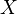defines a complex of presheaves with transfers, and hence we can define motivic cohomology ofas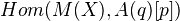, taken in the cat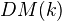or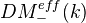I think. The main goal of the paper is to define a tensor triang cat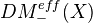such that the motivic cohomology ofcan be expressed as Hom from the unit object to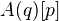in this cat. It seems like we always work with simplicial schemes over a perfect field.

<]]>
• ## Paper References

André, chapters 15-17.

<]]>

<]]>

<]]>

<]]>

<]]>

<]]>

<]]>

<]]>
• ## Some Research Articles

Huber: Realization of Voevodsky's motives (with a Corrigendum)

Spitzweck: Some constructions for Voevoedsky's triangulated category of motives

In the letter to Beilinson, Voevodsky formulates axioms for a homology theory. He considers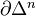as an (n-1)-dim sphere, write also S for the 1-dim sphere in this sense. Let Sch/k be the cat of separated schemes of finite type over a base k. Then a homological theory is a functor from Sch/k together with a family of natural isos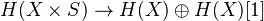. This functor should satisfy some conditions: Morally, homotopy invariance, MV exact triangle, an exact triangle for blowups, and transfer for flat finite morphisms. Get a 2-cat of homological theories over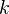. Examples: Algebraic K-th with rational coeffs, l-adic homology, Hodge homology ass to a complex embedding. Thm: There is an initial object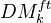in this cat, which we call the triang cat of eff mixed motives over k. Notion of reduced homological theory, and reduced motive of a scheme. Any motive in the above sense is of the form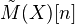, where we may assumeaffine and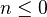. Tate object and comparison with K-theory. Bigger cat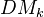which contains the previous as a full triang subcat, but admits a more explicit description rather than just the universal property. Can also be viewed as the closure of the previous, wrt direct sums and inductive limits. Need the h-topology, in particular coverings including surjective blowups, finite surjetive maps, etale coverings. Various filtrations on(homotopy canonical, geometrical, motivic canonical, weight). The weight filtr should be related to pure numerical motives.

<]]>
• ## Other Information

A fundamental idea in Voevodsky's thesis and in Homology of schemes I, is the homological cat of a site with interval. This might not be so much emphasized for example in MVW, but it seems very natural, and gives some intuition for the def of DM.

<]]>